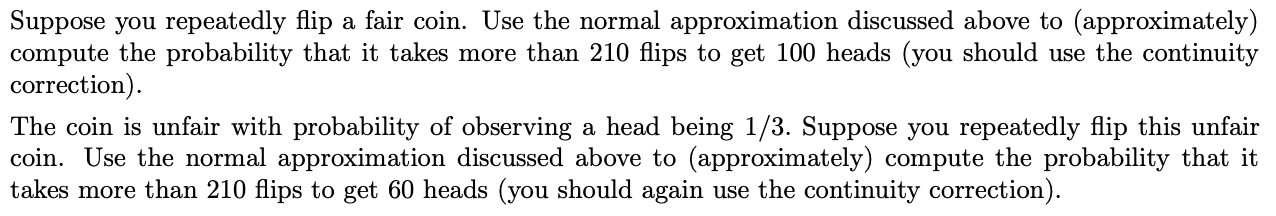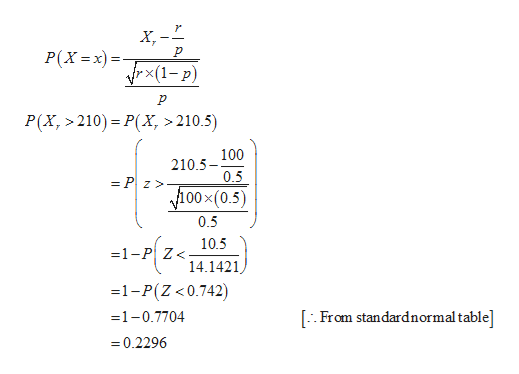# Suppose you repeatedly flip a fair coin. Use the normal approximation discussed above to (approximately)compute the probability that it takes more than 210 flips to get 100 heads (you should use the continuitycorrection).The coin is unfair with probability of observing a head being 1/3. Suppose you repeatedly flip this unfaircoin. Use the normal approximation discussed above to (approximately) compute the probability that ittakes more than 210 flips to get 60 heads (you should again use the continuity correction).

Question
16 viewshelp_outlineImage TranscriptioncloseSuppose you repeatedly flip a fair coin. Use the normal approximation discussed above to (approximately) compute the probability that it takes more than 210 flips to get 100 heads (you should use the continuity correction). The coin is unfair with probability of observing a head being 1/3. Suppose you repeatedly flip this unfair coin. Use the normal approximation discussed above to (approximately) compute the probability that it takes more than 210 flips to get 60 heads (you should again use the continuity correction). fullscreen
check_circle

Step 1

The probability that it takes more than 210 flips to get 100 heads is obtained below:

The required formula is,

Step 2

From the given information, flip a fair coin getting head or tail that is equally ...help_outlineImage TranscriptioncloseX, -* P(X =x) = rx(1- p) P(X,>210)= P(X, > 210.5) 100 210.5- 0.5 = P z> 100×(0.5) 0.5 10.5 =1-P Z< 14.1421 =1-P(Z <0.742) [:: From standardnormal table] =1-0.7704 = 0.2296 fullscreen

### Want to see the full answer?

See Solution

#### Want to see this answer and more?

Solutions are written by subject experts who are available 24/7. Questions are typically answered within 1 hour.*

See Solution
*Response times may vary by subject and question.
Tagged in

### Probability